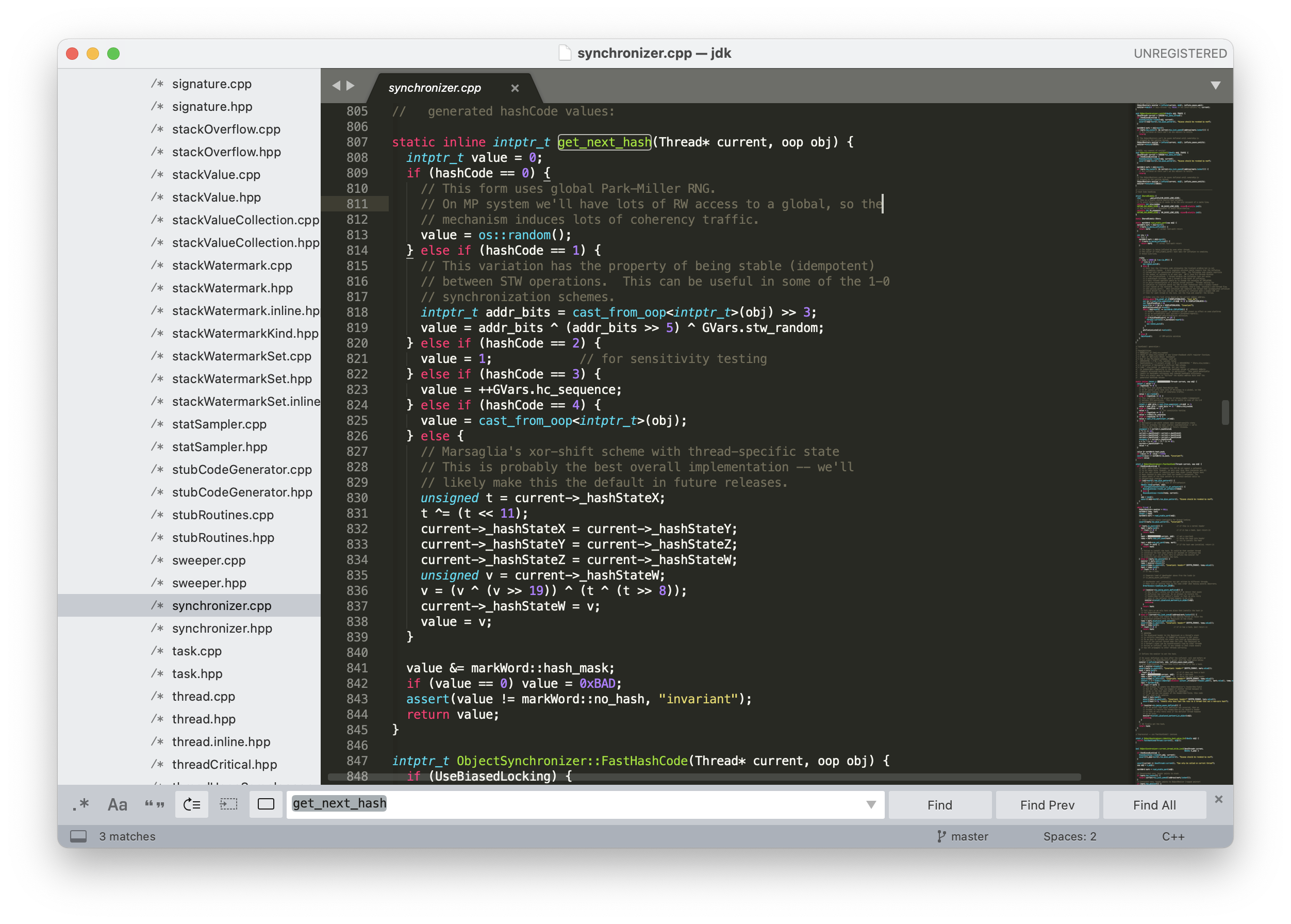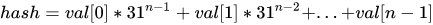为了彻底搞懂 hashCode，我连 JDK 的源码都没放过（建议收藏）

+关注继续查看

Object 类中就包含了 hashCode() 方法：

@HotSpotIntrinsicCandidate
public native int hashCode();GitHub 开源地址（欢迎 star）：https://github.com/itwanger/jmx-java

static final int hash(Object key) {
int h;
return (key == null) ? 0 : (h = key.hashCode()) ^ (h >>> 16);
}

public int hashCode() {
int h = hash;
if (h == 0 && value.length > 0) {
hash = h = isLatin1() ? StringLatin1.hashCode(value)
: StringUTF16.hashCode(value);
}
return h;
}
public static int hashCode(byte[] value) {
int h = 0;
int length = value.length >> 1;
for (int i = 0; i < length; i++) {
h = 31 * h + getChar(value, i);
}
return h;
}

public class Test {
public static void main(String[] args) {
Student s1 = new Student(18, "张三");
Map<Student, Integer> scores = new HashMap<>();
scores.put(s1, 98);
System.out.println(scores.get(new Student(18, "张三")));
}
}
class Student {
private int age;
private String name;
public Student(int age, String name) {
this.age = age;
this.name = name;
}
@Override
public boolean equals(Object o) {
Student student = (Student) o;
return age == student.age &&
Objects.equals(name, student.name);
}
}
1

null

HashMap 的 get() 方法会调用 hash(key.hashCode()) 计算对象的哈希值，虽然两个不同的 hashCode() 结果经过 hash() 方法计算后有可能得到相同的结果，但这种概率微乎其微，所以就导致 scores.get(new Student(18, "张三")) 无法得到预期的值 18。

@Override

public int hashCode() {

return Objects.hash(age, name);

}

Objects 类的 hash() 方法可以针对不同数量的参数生成新的 hashCode() 值。

public static int hashCode(Object a[]) {
if (a == null)
return 0;
int result = 1;
for (Object element : a)
result = 31 * result + (element == null ? 0 : element.hashCode());
return result;
}《Java 编程思想》这本圣经中有一段话，对 hashCode() 方法进行了一段描述。

static inline intptr_t get_next_hash(Thread* current, oop obj) {
intptr_t value = 0;
if (hashCode == 0) {
// This form uses global Park-Miller RNG.
// On MP system we'll have lots of RW access to a global, so the
// mechanism induces lots of coherency traffic.
value = os::random();
} else if (hashCode == 1) {
// This variation has the property of being stable (idempotent)
// between STW operations.  This can be useful in some of the 1-0
// synchronization schemes.
intptr_t addr_bits = cast_from_oop<intptr_t>(obj) >> 3;
} else if (hashCode == 2) {
value = 1;            // for sensitivity testing
} else if (hashCode == 3) {
value = ++GVars.hc_sequence;
} else if (hashCode == 4) {
value = cast_from_oop<intptr_t>(obj);
} else {
// Marsaglia's xor-shift scheme with thread-specific state
// This is probably the best overall implementation -- we'll
// likely make this the default in future releases.
unsigned t = current->_hashStateX;
t ^= (t << 11);
current->_hashStateX = current->_hashStateY;
current->_hashStateY = current->_hashStateZ;
current->_hashStateZ = current->_hashStateW;
unsigned v = current->_hashStateW;
v = (v ^ (v >> 19)) ^ (t ^ (t >> 8));
current->_hashStateW = v;
value = v;
}
if (value == 0) value = 0xBAD;
assert(value != markWord::no_hash, "invariant");
return value;
}

hashCode==0，调用操作系统 OS 的 random() 方法返回随机数。

hashCode == 1，在 STW（stop-the-world）操作中，这种策略通常用于同步方案中。利用对象地址进行计算，使用不经常更新的随机数（GVars.stw_random）参与其中。

hashCode == 2，使用返回 1，用于某些情况下的测试。

hashCode == 3，从 0 开始计算哈希值，不是线程安全的，多个线程可能会得到相同的哈希值。

hashCode == 4，与创建对象的内存位置有关，原样输出。

hashCode == 5，默认值，支持多线程，使用了 Marsaglia 的 xor-shift 算法产生伪随机数。所谓的 xor-shift 算法，简单来说，看起来就是一个移位寄存器，每次移入的位由寄存器中若干位取异或生成。所谓的伪随机数，不是完全随机的，但是真随机生成比较困难，所以只要能通过一定的随机数统计检测，就可以当作真随机数来使用。9555 014389 011269 0windows server 2008阿里云ECS服务器安全设置

9068 013252 04624 06792 07392 021971 054344 0

1084

0

《2021云上架构与运维峰会演讲合集》

《零基础CSS入门教程》

《零基础HTML入门教程》